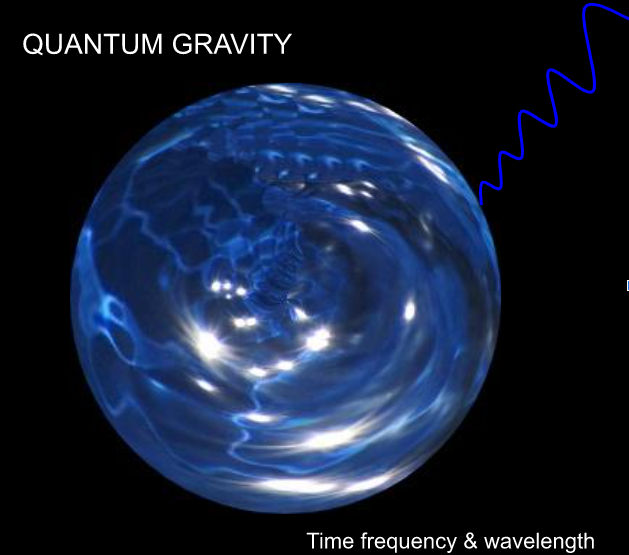top of page
Search
• Teslawaves

# Quantum gravity

The following hypothesis suggests that the universal constant big ‘G’ is the average value of nucleus gravity detectable at the atomic perimeter providing the illusion that nucleus gravity is very weak. The surface gravity of an atom's nucleus may be as strong as our sun's gravity that weakens very quickly to the value of big ‘G’ near the atom's perimeter. Indeed unleashing the potential of an atoms nucleus led to the creation of the atom bomb.

Newton's formula to determine N.t. gravity is the product of G times the mass, divided by the mass radius squared, or g = (G x M) / r2 . Where g is gravity, G the universal constant equal to (6.674×10 -11), M the mass of the sun and r the radius of the sun in metres. With the numbers inserted the sun's gravity is equal to + - 274m/s = (6.673 x 10 -11 x 1.989 x 10 30) / 695,700,000^2.

Teslawaves formula to determine Nucleus gravity is g(Q.t.) = (m/r^2), where g is gravity, m nucleus mass, r the radius of the nucleus, Q.t. Quantum time & N.t. Normal time. Note the actual radius is presumed but not known.

The following example will assume that the solar system is similar to an Oxygen atom with 8 protons and 8 neutrons surrounded by a sea of 8 electrons. In order to calculate nucleus gravity the formula g(Q.t.) = (m/r^2) = M (protons+neutrons) / r^2 is used. Where, g(Q.t.) is quantum gravity and ‘M’ equal to the total mass of protons & neutrons within the nucleus and (r) is the radius of the nucleus. When calculating nucleus gravity Big ‘G’ is not required because ‘G’ is equal to an atom's average perimeter gravity.

The total mass of eight protons plus eight neutrons multiplied by the mass of a proton is equal to about 2.68 x 10 -26 Kg = (1.672 x 10 -27 Kg x 16 ). The ‘proposed’ radius (r) of the Oxygen nucleus is equal to 9.889 x 10 -15 m. Note that in order to simplify the calculation neutrons which are marginally more massive than protons have been assigned the same mass as a proton. With the numbers inserted nuclei surface acceleration is equal to 274 Q.t.m per Q.t/s = (2.68 x 10 -26 Kg) / (9.889 x 10 -15 m)^2. And also equal to 274 N.t.m per N.t/s. However the strength of this strong N.t. gravitational force is only present over several tens of thousands of Quantum metres. One nuclei Q.t. metre is assumed to be in the range of about 1.38 x 10-23 of one Normal time metre = (9.89 x 10 -15 x 1.39 x 10 -09 ), equal to the diameter of the nucleus multiplied by the the diameter of the sun in metres by using the negative exponent to represent the number of metres across the radius of the nucleus.

At the atoms ‘detectable’ perimeter, approximately 2,023,000 nucleus radii, a figure based on the value of gravity at a distance from our star equal to 2,023,000 sun radii, about 54.5 light days, nucleus gravity is equal to big ‘G’ or 6.70 x 10 -11 m/s/s = 274m/s / (2,023,000)^2.

Gravity is therefore a very strong force within the atom but extremely weak at the atoms perimeter. And because gravity is cumulative the range of this ‘strong’ nucleus gravity can be extended into the N.t. dimension by adding more mass. So when calculating nucleus gravity the formula g = M (protons+neutrons) / r ^ 2 is used. But when calculating ‘Normal mass time’ gravity, Newton's formula of g = (G x M) / r^2 is used.

Gravity at the Quantum scale is extremely strong but weakens very quickly. Quantum time gravity per Quantum time second is the same strength as Normal time gravity per Normal time second but its ‘reach’ is very short. However sufficient mass can extend the mass radius extending the strength of gravity’s reach. Gravity can be both attractive and in the form of angular momentum, by creating a gravitational slingshot, also repulsive.

Quantum forces may all be manifestations of gravity, the same force which is multidimensional separated only by time and distance.More at Teslawaves.com